Home > GC > Chapter 6 > Lesson 6.1.2 > Problem6-14

6-14.
1. the triangles below. For each one, solve for x and name which tool you used. Show all work. Homework Help ✎

1.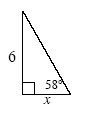2.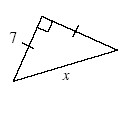3.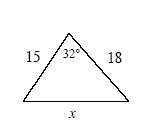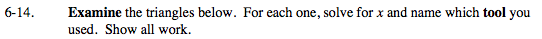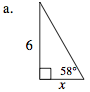Which trigonometric ratio can you use to solve for x?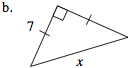What do the tick marks on the sides of the triangle mean?
How can you use that knowledge to solve for x?

Because of the marks, the lengths of the sides are the same.

Since this is a Right Isosceles triangle, use the Pythagorean Theorem.

x ≈ 9.9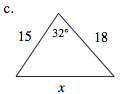Use the Law of Cosines to solve for x.

x ≈ 9.54